# Examples of State of Stress Civil Engineering (CE) Notes | EduRev

## Civil Engineering (CE) : Examples of State of Stress Civil Engineering (CE) Notes | EduRev

The document Examples of State of Stress Civil Engineering (CE) Notes | EduRev is a part of the Civil Engineering (CE) Course Advanced Solid Mechanics - Notes, Videos, MCQs & PPTs.
All you need of Civil Engineering (CE) at this link: Civil Engineering (CE)

Examples of state of stress
Specifying a state of stress means providing sufficient information to compute the components of the stress tensor with respect to some basis. As discussed before, knowing t(n) for three independent pairs of {t(n) , n} we can construct the stress tensor σ. Hence, specifying the set of pairs {(t(n) , n)} for three independent normal vectors, n, at a given point, so that the stress tensor could be uniquely determined tantamount to prescribing the state of stress. Next, we shall look at some states of stress.
The state of stress is said to be uniform if the stress tensor does not depend on the space coordinates at each time t, when the stress tensor is represented using Cartesian basis vectors.
If the stress tensor has a representation

σ = σn n,                            (4.29)

at some point, where n is a unit vector, we say it is in a pure normal stress state. Post-multiplying (4.29) with the unit vector n we find that

σn = σ(n  n)n = σ(n · n)n = σn =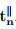(4.30)

Evidently, the traction is along (or opposite to) n. This stress σ characterizes either pure tension (if σ > 0) or pure compression (if σ < 0).

If we have a uniform stress state and the stress tensor when represented using a Cartesian basis is such that σxx = σ = const and all other stress components are zero, then such a stress state is referred to as uniaxial tension or uniaxial compression depending on whether σ is positive or negative respectively. This may be imagined as the stress in a rod with uniform cross-section generated by forces applied to its plane ends in the x − direction. However, recognize that this is not the only system of forces that would result in the above state of stress; all that we require is that the resultant of a system of forces should be oriented along the ex direction.

If the stress tensor has a representation

σ = σ(n  n + mm),                                                        (4.31)

at any point, where n and m are unit vectors such that n · m = 0, then it is said to be in equibiaxial stress state. On the other hand, if the stress tensor has a representation

σ = τ (n  m + m  n),                                                        (4.32)

at any point, where n and m are unit vectors such that n · m = 0, then it is said to be in pure shear stress state. Post-multiplying (4.32) with the unit vector n we obtain

σn = τ (n  m + m  n)n
= τ [(m · n)n + (n · n)m] = τm =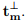(4.33)

Evidently,is tangential to the surface whose outward unit normal is along (or opposite to) n.

More generally, if the stress tensor has a representation

σ = σ1nn + σ2mm,                                                   (4.34)

at any point, where n and m are unit vectors such that n · m = 0, then it is said to be in plane or biaxial state of stress. That is in this case one of the principal stresses is zero. A general matrix representation for the stress tensor corresponding to a plane stress state is: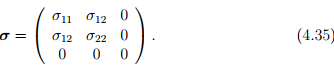Here we have assumed that e3 is a principal direction and that there exist no stress components along this direction. We could have assumed the same with respect to any one of the other basis vectors. A plane stress state occurs at any unloaded surface in a continuum body and is of practical interest.
Next, we consider 3D stress states. Analogous to the equibiaxial stress state in 2D, if the stress tensor has a representation

σ = p1,                                                                          (4.36)

at some point, we say that it is in a hydrostatic state of stress and p is called as hydrostatic pressure. It is just customary to consider compressive hydrostatic pressure to be positive and hence the negative sign. Post-multiplying (4.36) by some unit vector n, we obtain

σn = (−p1)n = −pn =.                                             (4.37)

Thus, on any surface only normal traction acts, which is characteristic of (elastic) fluids at rest that is not able to sustain shear stresses. Hence, this stress is called hydrostatic. Any other state of stress is called to be triaxial stress state. Many a times the stress is uniquely additively decomposed into two parts namely an hydrostatic component and a deviatoric component, that is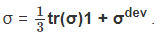(4.38)

Thus, the deviatoric stress is by definition,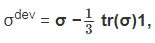(4.39)

and has the property that tr(σdev) = 0. Physically the hydrostatic component of the stress is supposed to cause volume changes in the body and the deviatoric component cause distortion in the body. (Shear deformation is a kind of distortional deformation.)

Offer running on EduRev: Apply code STAYHOME200 to get INR 200 off on our premium plan EduRev Infinity!

## Advanced Solid Mechanics - Notes, Videos, MCQs & PPTs

42 videos|61 docs

,

,

,

,

,

,

,

,

,

,

,

,

,

,

,

,

,

,

,

,

,

;